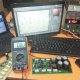Breaking News
Home / making transformer / How to make battery charger?

# How to make battery charger?

Here in this post, we can learn how to make Battery charger for any battery. How to make battery charger

we are going to make 0-12-24-36 voltage battery charger. we need to charge 3 battery together. normally we can buy many kinds of battery. many kinds of the ampere. but we can’t buy the charger for that battery according to our battery ampere. for this, we had to make the charger according to our needs.

Let’s go to make a battery charger: here we have a 100 amperes 12 voltage battery.

Let’s go to make a charger for this 12 voltage 100 amperes Battery. first, we have to decide how many hours we can charge the battery. if we decide we can charge the battery 12 hours. so our battery is 100 amp so 100/12=8.3 ampere and 12 voltage need to charge the Battery in 12 hours. or we 5 amperes and 12 voltage then 100/5=20 hours. 20 hours need if we use 5 amperes 12 voltage charger.

ok, we will make a charger for 20 hours. so we need 5 amperes 12 voltage. We know that Voltage X Ampere= Watts. 12X5=60 Watts. our transformer will be 60 Watts.

wire gauge and ampere.

Gauge     –      Ampere

7              –        44.2

8              –        33.3

9              –        26.5

10            –        21.2

11            –        16.6

12            –        13.5

13            –        10.5

14            –          8.3

15            –          6.6

16            –          5.2

17            –          4.1

18            –          3.2

19            –          2.6

20            –          2.0

21            –          1.6

22            –          1.2

23            –          1.0

24            –          0.8

25            –          0.6

26            –          0.5

27            –          0.4

28            –          0.3

29            –          0.29

30            –          0.22

## The primary binding:

We have to make 60 watts transformer. if our AC voltage is 220 then 60/220=0.27. so 0.27-ampere wire need for the primary binding we can use the 18 number 0.27 ampere wire is 29 gauge number wire.

## The secondary binding:

we need 5 amperes so we have to use 16 number Gauge wire.

Let’s go to watch how to make transformer?